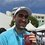# Y=mx+c

In this chapter we’re going to be looking at y=mx+c graphs. We shall explore the effects of m and c on the line in the y=mx+c equation. We shall then explore how to find the equation of a line drawn on a graph paper.

Example

Plot the graph of y=2x+1 To plot a graph we usually draw a table and then try to find the value of y for the chosen x values. You need to editing and make sure that the chosen values are close to each other, for example 1 to 10. Below is the table that will help us plot the graph of y=2x+1.

x y 0 1 1 3 2 5 3 7 4 9

Now we can plot these values on the graph as shown below. Now we join the plotted points with a single straight line.

M and C in y=mx+c In the equation y=mx+c m changes the steepness of the line. The following shows a graph which has lines whose m values have been changed.

The C in y=mx+c changes where the line intercepts the y-axis by moving it down or upwards. The following graph has lines whose C are different.

Gradient

Above we’ve seen that as the m value changes the steepness of the line changes. The steepness of the line in mathematics is known as gradient

We find the gradient on a straight line by dividing the number of units covered by a line across with the number of units covered by the line down. The following is the line of y=½x+c

One divided by two gives ½, this is what is known as the gradient of the line.

Finding the equation of a straight line Below we can see a graph whose equation is unknown.

Since the above is a straight line. The equation will be in the form of y=mx+c. We need to find the values of m and c in the equation. We know that the c value indicates where the line intercepts the y-axis so;

We know that m is the gradient of the line and we find this by dividing the upwards coverage with the downwards coverage;Note by Eddie Hartley
2 years, 6 months ago

This discussion board is a place to discuss our Daily Challenges and the math and science related to those challenges. Explanations are more than just a solution — they should explain the steps and thinking strategies that you used to obtain the solution. Comments should further the discussion of math and science.

When posting on Brilliant:

• Use the emojis to react to an explanation, whether you're congratulating a job well done , or just really confused .
• Ask specific questions about the challenge or the steps in somebody's explanation. Well-posed questions can add a lot to the discussion, but posting "I don't understand!" doesn't help anyone.
• Try to contribute something new to the discussion, whether it is an extension, generalization or other idea related to the challenge.
• Stay on topic — we're all here to learn more about math and science, not to hear about your favorite get-rich-quick scheme or current world events.

MarkdownAppears as
*italics* or _italics_ italics
**bold** or __bold__ bold
- bulleted- list
• bulleted
• list
1. numbered2. list
1. numbered
2. list
Note: you must add a full line of space before and after lists for them to show up correctly
paragraph 1paragraph 2

paragraph 1

paragraph 2

[example link](https://brilliant.org)example link
> This is a quote
This is a quote
    # I indented these lines
# 4 spaces, and now they show
# up as a code block.

print "hello world"
# I indented these lines
# 4 spaces, and now they show
# up as a code block.

print "hello world"
MathAppears as
Remember to wrap math in $$ ... $$ or $ ... $ to ensure proper formatting.
2 \times 3 $2 \times 3$
2^{34} $2^{34}$
a_{i-1} $a_{i-1}$
\frac{2}{3} $\frac{2}{3}$
\sqrt{2} $\sqrt{2}$
\sum_{i=1}^3 $\sum_{i=1}^3$
\sin \theta $\sin \theta$
\boxed{123} $\boxed{123}$

## Comments

There are no comments in this discussion.

×

Problem Loading...

Note Loading...

Set Loading...